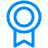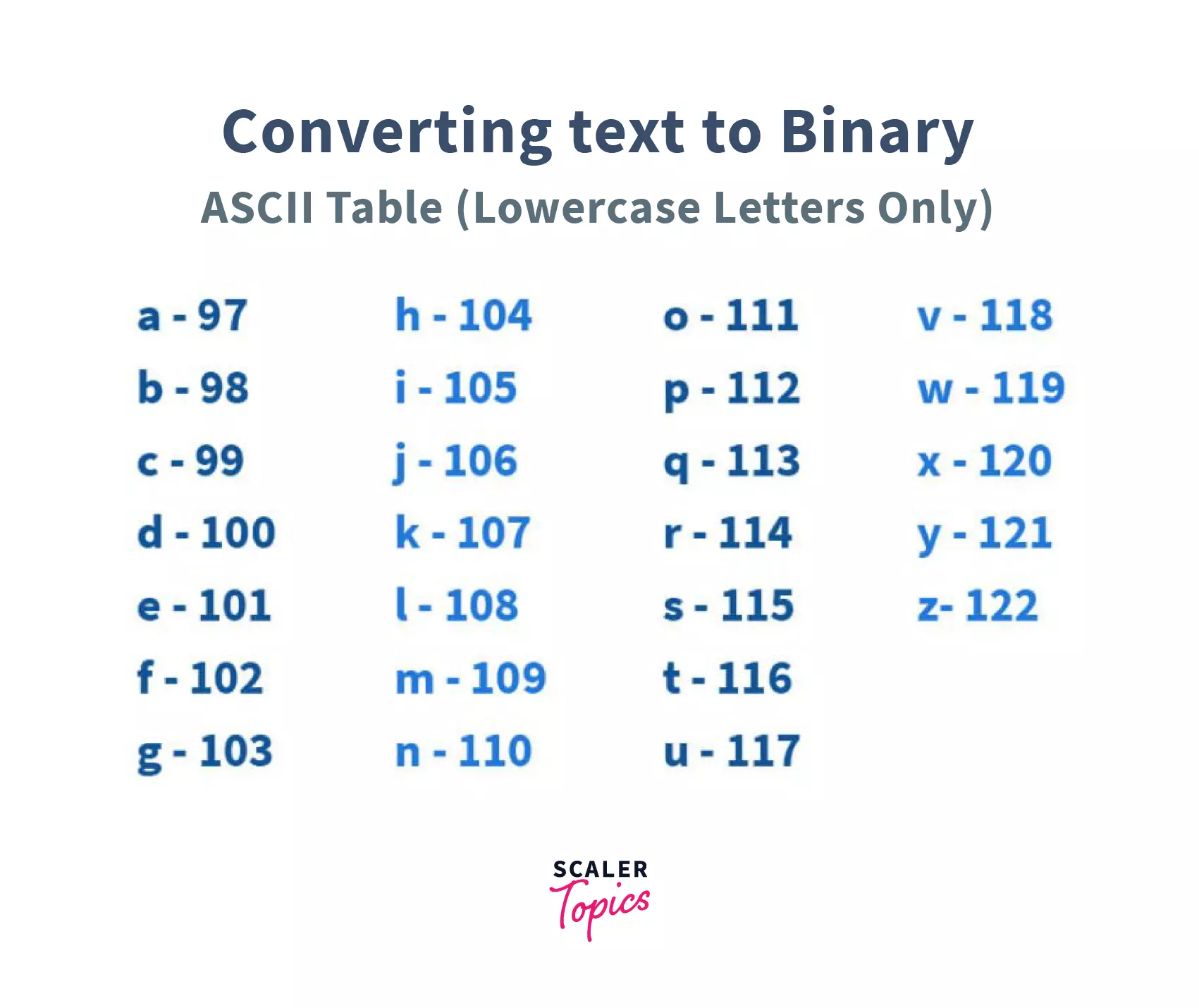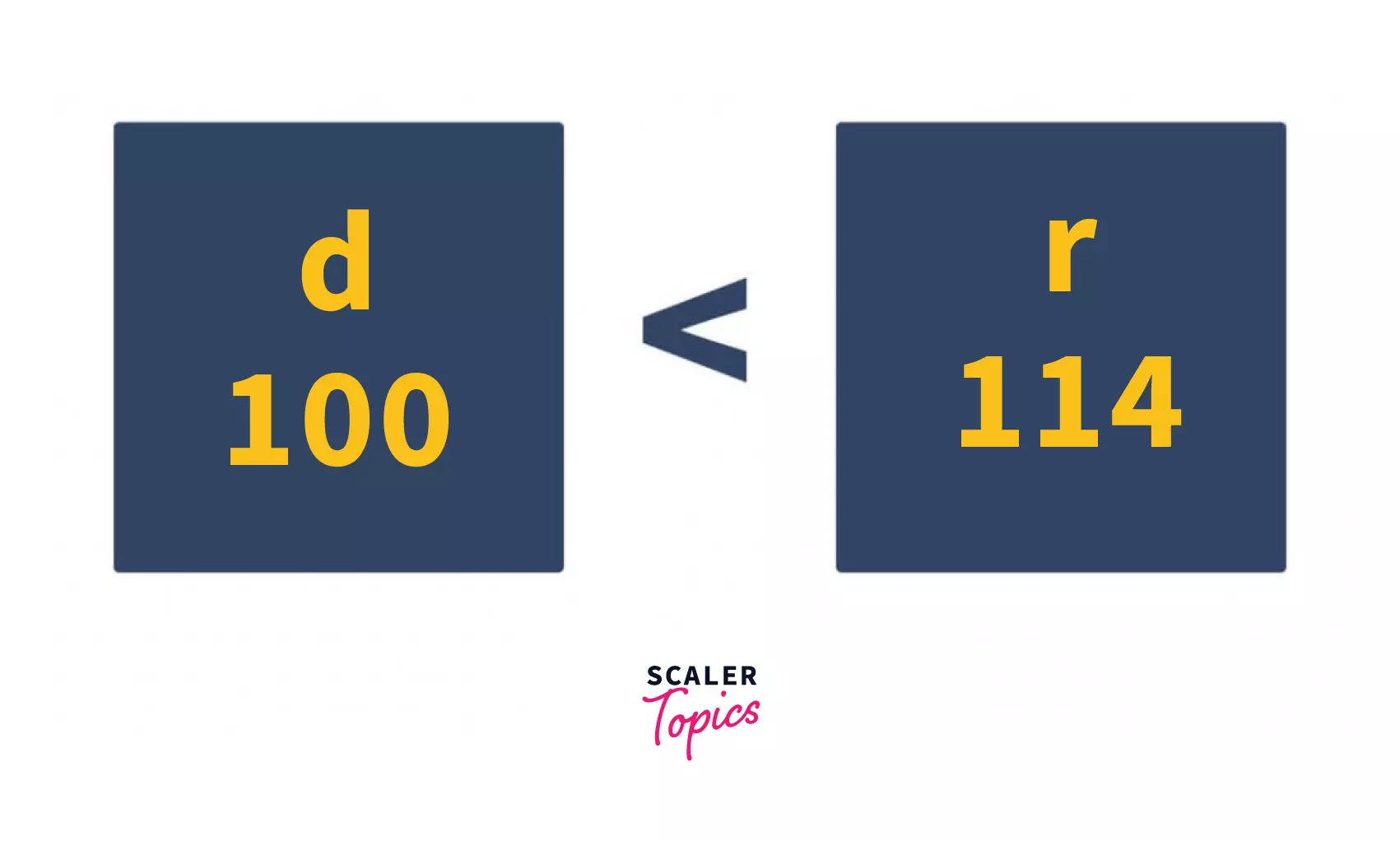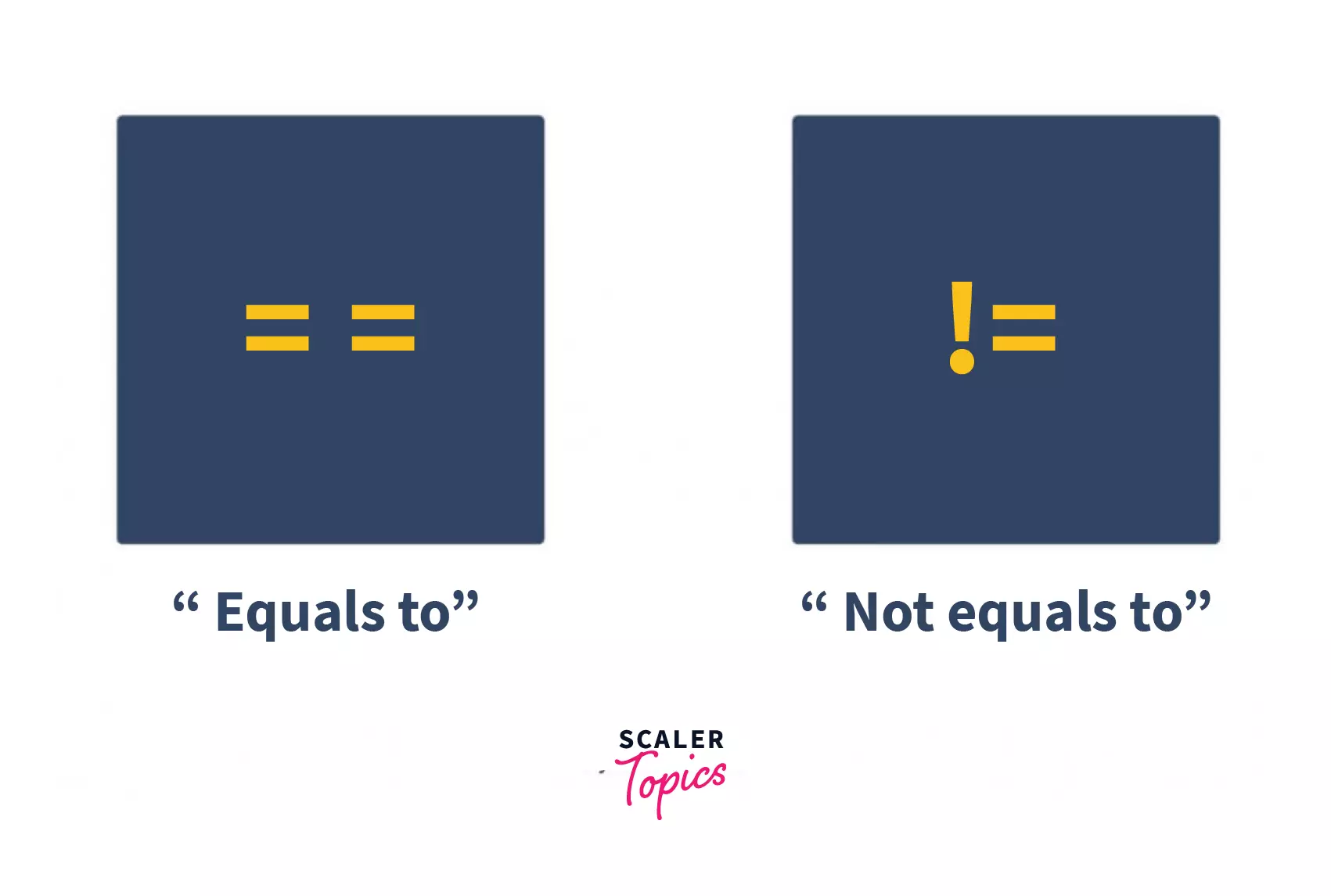Certificate

### Learn on Scaler Topics and get certified.# Comparing Two Strings in C++

Learn 3 Methods to Compare Two Strings in C++.

Updated - 12 May 20227 mins readPublished : 16 Jul 2021
Published : 16 Jul 2021Challenge Inside! : Find out where you stand! Try quiz, solve problems & win rewards!

## Overview

String comparison is a very common scenario in programming. Most string comparison algorithms compare the strings character by character. C++ provides us with built-in operators like == and != to compare the strings along with strcmp and compare functions.

## Scope

• This article covers the intuition behind string comparison.
• It covers different ways to compare two strings in C++.

## Introduction

There are certain use cases in programming where we feel the need to compare two strings. For example, consider a website having two fields, ‘Email’ & ‘Confirm Email’. Then, we would have to use some string comparison to confirm whether both the entered email strings are equal or not.In this article, we shall learn how to compare two strings in C++, but before that, let's try to understand the intuition behind comparing two strings.

### Understanding the Intuition/Algorithm behind Comparing Two Strings:

Before we proceed further, it’s important to reiterate and reinforce the fact that in classical computing, everything essentially is a number. Therefore, characters that constitute together to make a string are numerical entities.

Every character has something called an ASCII value. Hence characters can be compared easily.Let’s understand the intuition behind the comparison between two strings.

First, momentarily picture this. While shopping on e-commerce websites like Amazon or Flipkart, you might have often keyed in your debit or credit card number – while checking out.

How do you check whether what you have filled in is the correct number?

The optimal way could be to go digit by digit, from left to right. Well, that’s just how strings are compared as well.

Let's see this in action.

Consider two strings of equal length here: String #1: “Scaler” String #2: “Scaled”We start comparing strings from left to right, character by character, just like you would have checked your debit card number.

But before we even dive into applying our brain cells in deducing whether strings are equal or not, focus on the string lengths.

See it this way. If the debit card number you have entered is less than 16 digits, you don’t even have to evaluate whether what you have entered is right or wrong.

It’s simply wrong!

Similarly, in terms of strings, if there is a length mismatch between the first string & second string, there’s no way they are equal. You can confidently say that the strings are unequal.

But if the strings are of equal length, which in our case is (Scaler & Scaled, in case any of you folks forgot ), we continue to march forward.

1. The first character at index 0 in both strings is ‘S’. Since ‘S’ is equal to ‘S’, we continue.
2. The second character in both strings is the character ‘c’. Again, since ‘c’ is equal to ‘c’, we continue.
3. The third character in both strings is equal to ‘a’. Continue.
4. The fourth character is equal since ‘l’ is equal to ‘l’.
5. Character ‘e’ is equal to ‘e’. Hence the fifth characters are equal. We continue.
6. The sixth character in the string #1 and string #2 present an exception. The sixth character in the string #1 is ‘r’, while the sixth character in the string #2 is ‘d’.Note the usage of the term lexicographically here, which essentially means ‘in dictionary order’. Thus it would be equally correct to say that string ‘Scaler’ is higher in dictionary order when compared to the string ‘Scaled’.

## Three Ways to Compare Strings in C++

There are three ways to compare strings in C++. Let’s take a look at each one of them one by one.

## 1. Comparing Two Strings Using strcmp() Function in C++

strcmp() is a C library function that compares two strings lexicographically.

It is an inbuilt function in C++ String.

Syntax:

``````int strcmp(const char* firstString, const char* secondString)
``````

strcmp() function takes two strings (character arrays) as arguments and returns: 0, if both the strings are equal i.e. firstString is lexicographically equal to secondString.

As verbose as it might sound, as stated, lexicographically means “in alphabetical order”.

As a rule of thumb, if a word in a regular Oxford dictionary comes before another, the first word is called lexicographically smaller than the second word.

If they are to appear “together” in a copy of the Oxford dictionary, they are said to be lexicographically equal.

There is no point in guessing that if a word appears after another word in the dictionary, it is lexicographically greater than the second word.

B. Greater than 0, if firstString is lexicographically greater than secondString.

C. Less than 0, if firstString is lexicographically smaller than secondString.

Consider the following piece of code to gain more clarity on the usage of the strcmp() function in C++:

``````const char * firstString = “Scaler”;
const char * secondString = “Scaled”;

int equalOrNot = strcmp(firstString, secondString);

if (equalOrNot == 0) {
cout << firstString << “and” << secondString << “are lexicographically equal strings” << ‘\n’;
} else {
cout << firstString << “and” << secondString << “are lexicographically unequal strings” << ‘\n’;
}
``````

Output:

``````Scaler and Scaled are lexicographically unequal strings.
``````

## 2. Comparing Two Strings in C++ Using compare() Function

compare() is a function defined in the standard library of C++ to compare two strings.

Syntax:

``````int compare(const string& secondString) const;
``````

compare() function in C++ gives broadly two classes of outputs when comparing strings:

A. Class #1: equal to 0 ( == 0 )

Zero is returned when the strings being compared are lexicographically equal.

B. Class #2: not equal to 0 ( != 0 )

compare() function in C++ returns non-zero values when the strings being compared are lexicographically unequal

There are further two sub-classes of outputs for non-zero output returned by the compare() function:

1. Greater than 0 ( > 0): this is returned when the first string is lexicographically greater than the second string
2. Less than 0 ( < 0 ): this is returned when the first string is lexicographically smaller than the second string

Let’s understand this better using a code example in C++:

``````string firstString = “Scaler”;
string secondString = “Scaled”;

int equalOrNot = firstString.compare(secondString);

if (equalOrNot == 0) {
cout << “String 1 & String 2 are equal” << ‘\n’;
} else if (equalOrNot > 0) {
cout << “String 1 is lexicographically greater than String 2” << ‘\n’;
} else {
cout << “String 1 is lexicographically smaller than string 2” << ‘\n’;
}
``````

Output:

``````String 1 is lexicographically greater than String 2
``````

## 3. Comparing Two Strings in C++ Using C++ Relational OperatorsApart from the inbuilt functions of strcmp() & compare(), C++ also offers a vanilla way of comparing two strings using relational operators ( ==, != )

You might have observed that we used the ‘==’ relational operator many times above.

E.g., when we use equalOrNot == 0 in our code examples above, we are simply comparing whether the value of our equalOrNot variable is equal to 0.

Therefore, the ‘==’ operator is used to compare two entities (strings or integers, for instance) to see whether they are equal in value. It’s undoubtedly one of the easiest ways to compare two strings in C++. There are two kinds of relational operators we wish to highlight here:

A. ‘==’ relational operator or “equals to” relational operator

B. ‘!=’ relational operator or “not equals to” relational operator

Let’s further understand comparing strings using these relational operators via a code example in C++:

A. Using ‘==’ (equals to) relational operator in C++:

``````string firstString = “Scaler”;
string secondString = “Scaled”;

if (firstString == secondString) {
cout << “String 1 is EQUAL to String 2” << ‘\n’;
} else {
cout << “String 1 is NOT EQUAL to String 2” << ‘\n’;
}
``````

Output:

``````String 1 is NOT EQUAL to String 2
``````

B. Using ‘!=’ (not equals to) relational operator in C++

``````string firstString = “Scaler”;
string secondString = “Scaled”;

if (firstString != secondString) {
cout << “String 1 is NOT EQUAL to String 2” << ‘\n’;
} else {
cout << “String 1 is EQUAL to String 2” << ‘\n’;
}
``````

Output:

``````String 1 is NOT EQUAL to String 2
``````

Note how even if the way of writing the conditions in code changes, the outputs remain unchanged in the above programs (as “Scaler” and “Scaled” strings are indeed unequal).

## Conclusion

In this article, we learned three ways to compare strings in C++, using:

• strcmp() inbuilt function
• compare() inbuilt function, and
• C++ relational operators ( ‘==’, ‘!=’)
Challenge Time!Time to test your skills and win rewards! Note: Rewards will be credited after the next product update.
Free Courses by top Scaler instructors